Open in App
Not now

# Trapezoidal Rule

• Difficulty Level : Medium
• Last Updated : 24 Jun, 2021

Riemann’s sums are used to approximate an area under the curve. The area under the curve is divided into rectangles, then the area of individual rectangles is calculated and their sum gives the total area. This method of approximation can also be used to arrive at the definition of the integrals. A similar approach is used by the trapezoidal rule. In this rule, the area under the curve is divided into many trapezoids whose area is then calculated and added to get an approximation of the whole area.

### Trapezoidal Sums

This rule is one of the most important rules in the theory of integration. Any area that is to be calculated is divided into many parts. As the name suggests, the area this time is divided into a trapezoidal shape. For a function f(x), the area enclosed by the function and the x-axis is given in the figure below. The goal is to calculate the area between x = a to x = b.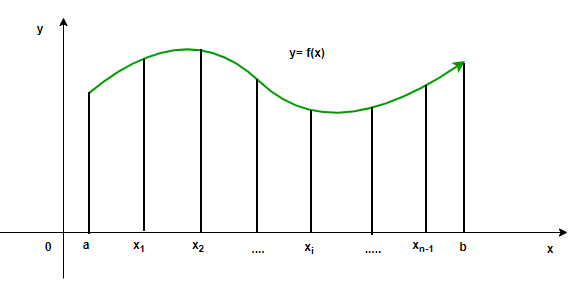Now, let’s divide the area under the curve between the limits into different trapezoids.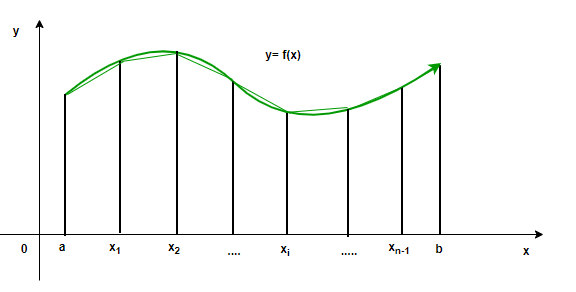Notice that there is still some area that is remaining. This means that this rule is an approximation, but the difference between the actual area and the approximated area decreases as the number of trapezoids increases. Theoretically, when the number of trapezoids reaches infinity, the approximated area becomes equal to the actual area.

Consider the function f(x) given in the figure above. Let f(x) be continuous in the interval. This interval is divided into n equal intervals, then the width of each interval becomes,Such that,

a = x0 < x1 < x2 < …. xn = b

We know the area for a trapezoid =So, the total area of the function under this approximation will become,Rearranging the above equation,Now, as the number of trapezoids increases, the sum becomes closer to the current area.Here,and### Summation Notation of Trapezoidal Rule

We know that the area of a trapezoid is basically the average of the lengths of the parallel sides multiplied by the height. So, in this case, consider a trapezoid for the ith interval,Since the total area is the sum of all the areas,

A = A1 + A2 + ….+ An

A =⇒A =This is called the sigma notation of the trapezoid sums.

### Riemann Sums

Just like the previous case, Riemann sums up work on the idea of diving the area under the curve into different rectangular parts. As the number of rectangles increases, the area becomes closer and closer to the current area. In the figure shown below, there is a function f(x). The area under this function is divided into many rectangles. The total area under the curve is the sum of the areas of all the rectangles.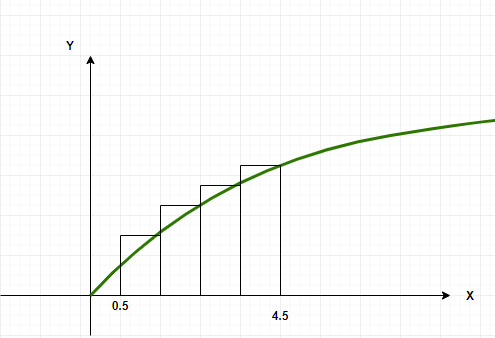Notice that here, the right end of the rectangles touch the curve. This is called right-Riemann sums. In another case, when the left end of the rectangles touches the curve, they are called left Riemann sums.  The figure below shows an example of the left-Riemann sum.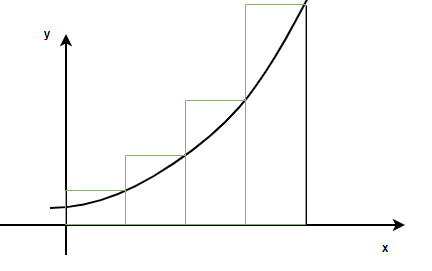Let’s sayis the interval width and n is the number of intervals as declared above. Then the sum is given by,### Middle Point Sums – summation notation

In the Riemann sums, either the left-end or the right end of the rectangle touches the curve. In this case, the middle point of the rectangle touches the curve. Everything else is the same as Riemann sums. The figure below shows the function f(x) and different rectangles in the middle point sums.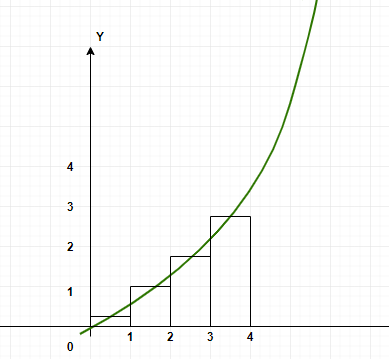Let’s say Ai denotes the area of the ith rectangle. The area of this rectangle in this case will be,Now, the total area in the summation notation will be,Let’s see some sample problems related to the trapezoid rule.

### Sample Problems

Question 1: Find the area enclosed by the function f(x) between x = 0 to x = 4 with 4 intervals.

f(x) = 4

Solution:

Here a = 0, b = 4 and n = 4.The trapezoidal rule for n = 4 is,Substituting the values in this equation,Question 2: Find the area enclosed by the function f(x) between x = 0 to x = 3 with 3 intervals.

f(x) = x

Solution:

Here a = 0, b = 3 and n = 3.The trapezoidal rule for n = 3 is,Substituting the values in this equation,Question 3: Find the area enclosed by the function f(x) between x = 0 to x = 2 with 2 intervals.

f(x) = 2x

Solution:

Here a = 0, b = 2 and n = 2.The trapezoidal rule for n = 2 is,Substituting the values in this equation,Question 4: Find the area enclosed by the function f(x) between x = 0 to x = 3 with 3 intervals.

f(x) = x2

Solution:

Here a = 0, b = 3 and n = 3.The trapezoidal rule for n = 3 is,Substituting the values in this equation,Question 5: Find the area enclosed by the function f(x) between x = 0 to x = 4 with 4 intervals.

f(x) = x3 + 1

Solution:

Here a = 0, b = 4 and n = 4.The trapezoidal rule for n = 4 is,Substituting the values in this equation,Question 6: Find the area enclosed by the function f(x) between x = 0 to x = 4 with 4 intervals.

f(x) = ex

Solution:

Here a = 0, b = 4 and n = 4.The trapezoidal rule for n = 4 is,Substituting the values in this equation,Question 7: Find the area enclosed by the function f(x) given below between x = 0 to x = 10 with 5 intervals.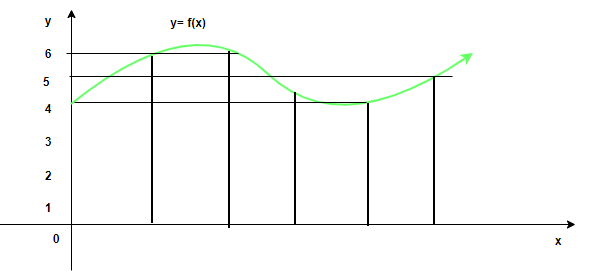Solution:

Here a = 0, b = 10 and n = 5.The trapezoidal rule for n = 5 is,From the graph, the values of the function can be inferred,My Personal Notes arrow_drop_up
Related Articles